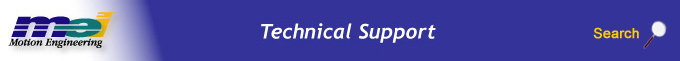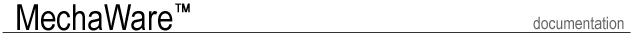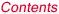.Select a Step ......................................................... Introduction Features User Interface Requirements Types of MechaWare Blocks Block Simulink Library Matlab Utility Software Tools Case Study Tutorial

Types of MechaWare Blocks

There are four types of MechaWare blocks: I/O, Computational, Data File, and Advanced.

Input/Output Blocks

Input / Output blocks are used to pass data to and from the Filter Objects. The filter algorithm can read data from any Axis Object and can write data to any Motor Object. The ability to access multiple data locations with the Input/Output blocks makes the design of MIMO (multi Input, multi Output) control algorithms possible. I/O blocks require a specific format for data entry, the format is the block tag followed by the desired axis number.

 Input/Output Blocks Block Type Tag Actual Position Input ACTUAL Axis Input AXIS Command Position Input COMMAND Error Input ERROR Constant Input CONSTANT TC Vel Input AXIS TC Acc Input AXIS Noise Input NOISE Scope Output SCOPE Output Output OUTPUTIn the example above, the block uses the actual position from Axis 3 as feedback.

Computation Blocks

Computational blocks support basic mathematical operations, biquad filters, and matrix computation. Each block will generate a single output from a single or multiple inputs, depending on the block's functionality.

 Computation Blocks Block Tag Use Parameters Gain GAIN Constant gain K Matrix Gain MATRIXGAIN Vector product with sum Length, Coeff[] Differentiator DIFF Derivative with smoothing Ksm Integrator PID Integration Ki PID PID Proportional, Integral, Derivative Kp, Ki, Kd, Imax, Drate PIV PIV Proportional, Integral, Velocity Feedforward FF Four types of feedforward Kaff, Kvff, Kpff, Kfff BiQuad BIQ5 Double precision observer Length, Coeff[] Sp_BiQuad BIQ Single precision observer Length, Coeff[] Observer OBS2 Double precision observer Length, Coeff[] Observer OBS Single precision observer Length, Coeff[] Clip CLIP Clip to limits Limit, Offset Sum SUM Multi-input sum nSum, Coeff[] Multiplication MULT Multi-input product nMult Division DIV Multi-input quotient nDiv Sine SIN Sine None Cosine COS Cosine None

Biquad and Observers Blocks are defined in two ways. In the MATLAB workspace they are defined by a MechaWare biquad/observer structure. This structure is then used to create biquad and observer text files that are used to load block parameters to the ZMP or XMP controller.

The observer and the biquad blocks are defined in the discrete time domain. Mechaware structures include z-transform and difference equation descriptions of the blocks.

Z DomainDifference EquationsBiquad Structure Element Description OBS.b B(z): transfer function numerator OBS.a A(z): transfer function denominator OBS.A State transition matrix OBS.B Input state matrix OBS.C Output coupling matrix OBS.D Input to Output coupling matrix OBS.Ts Sample Rate OBS.sys Matlab LTI object

Observer Structures

Z DomainDifference EquationsObserver Structure Element Description OBS.b1 B(z): ufb(z)/u(z) numerator OBS.a1 A(z): ufb(z)/u(z) denominator OBS.b2 B(z): ufb(z)/ymeas(z) numerator OBS.a2 A(z): ufb(z)/ymeas(z) denominator OBS.A State transition matrix OBS.B Input state matrix OBS.C Output coupling matrix OBS.D Input to Output coupling matrix OBS.Ts Sample Rate OBS.sys Matlab LTI object